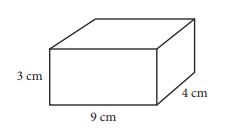Scan QR code or get instant email to install app

Question:

# The surface area of a right rectangular prism can be found by finding the sum of the area of each of the faces of the prism. What is the surface area of a right rectangular prism with length 4 centimeters (cm), width 9 cm, and height 3 cm? (Area of a rectangle is equal to length times width.)A 150 cm^2.
explanation

The surface area of the rectangular prism is the total area of each of the faces of the prism and can be written as
2(length × width) + 2(height × width) + 2(length × height),
which is:
2(4 cm × 9 cm) + 2(3 cm × 9 cm) + 2(4 cm × 3 cm), or 150 cm^2.